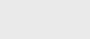3年前 (2020-10-17) |   抢沙发  1206

[收起] 文章目录

## 用递归反转序列

``````/∗∗ Reverses the contents of subarray data[low] through data[high] inclusive. ∗/
public static void reverseArray(int[ ] data, int low, int high) {
if (low < high) { // if at least two elements in subarray
int temp = data[low]; // swap data[low] and data[high]
data[low] = data[high];
data[high] = temp;
reverseArray(data, low + 1, high − 1); // recur on the rest
}
}``````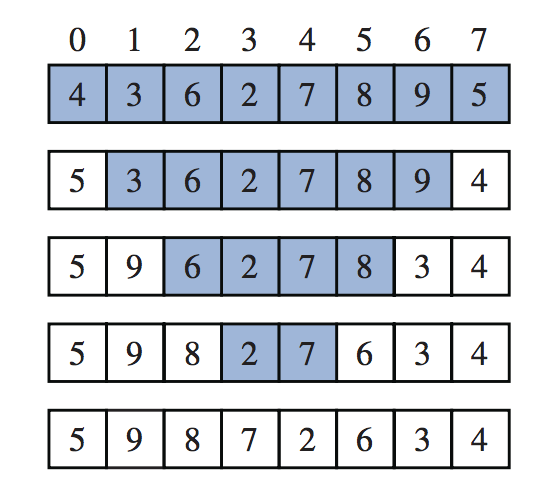## 计算幂的递归算法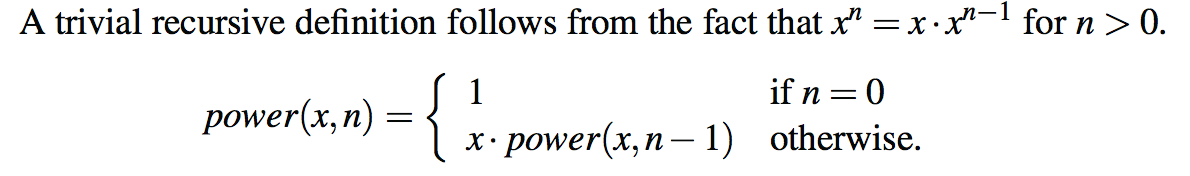``````/∗∗ Computes the value of x raised to the nth power, for nonnegative integer n. ∗/
public static double power(double x, int n) {
if (n == 0)
return 1;
else
return x ∗ power(x, n−1);
}``````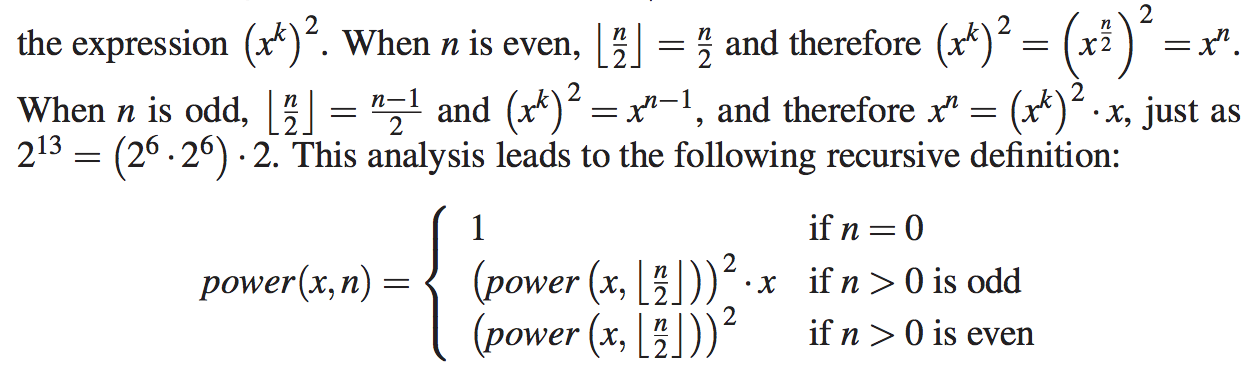``````/∗∗ Computes the value of x raised to the nth power, for nonnegative integer n. ∗/
public static double power(double x, int n) {
if (n == 0)
return 1;
else {
double partial = power(x, n/2); // rely on truncated division of n
double result = partial ∗ partial;
if (n % 2 == 1) // if n odd, include extra factor of x
result ∗= x;
return result;
}
}``````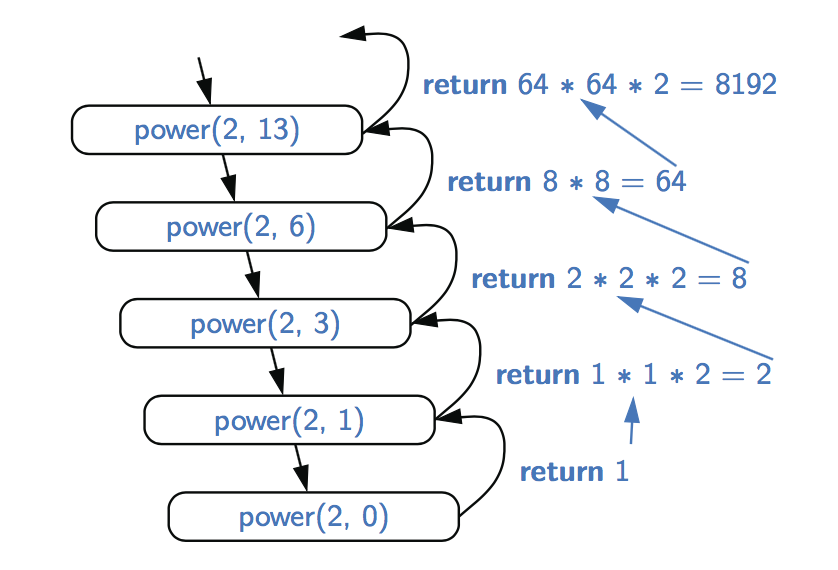## 二进制递归

``````/∗∗ Returns the sum of subarray data[low] through data[high] inclusive. ∗/
public static int binarySum(int[ ] data, int low, int high) {
if (low > high) // zero elements in subarray
return 0;
else if (low == high) // one element in subarray
return data[low];
else {
int mid = (low + high) / 2;
return binarySum(data, low, mid) + binarySum(data, mid+1, high);
}
}``````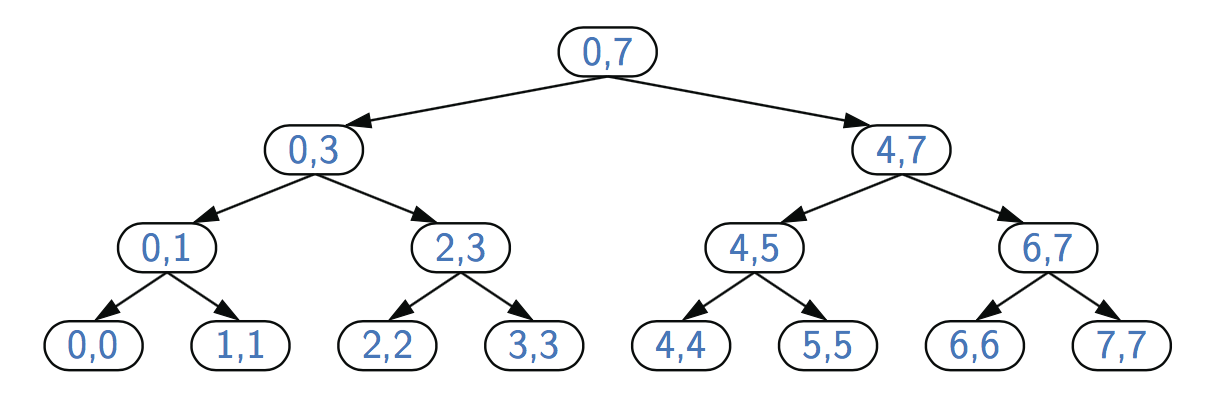## 多重递归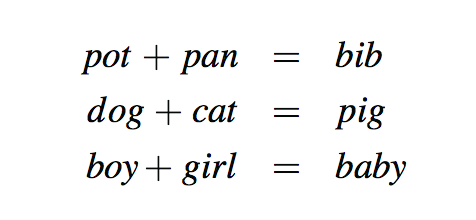1. 递归生成k−1元素序列
2. 在每个这样的序列中附加一个尚未包含在其中的元素

``````Algorithm PuzzleSolve(k, S, U):
Input: An integer k, sequence S, and set U
Output: An enumeration of all k-length extensions to S using elements in U
without repetitions
for each e in U do
Add e to the end of S
Remove e from U {e is now being used}
if k = = 1 then
Test whether S is a configuration that solves the puzzle
if S solves the puzzle then
add S to output {a solution}
else
PuzzleSolve(k−1, S, U) {a recursive call}
Remove e from the end of S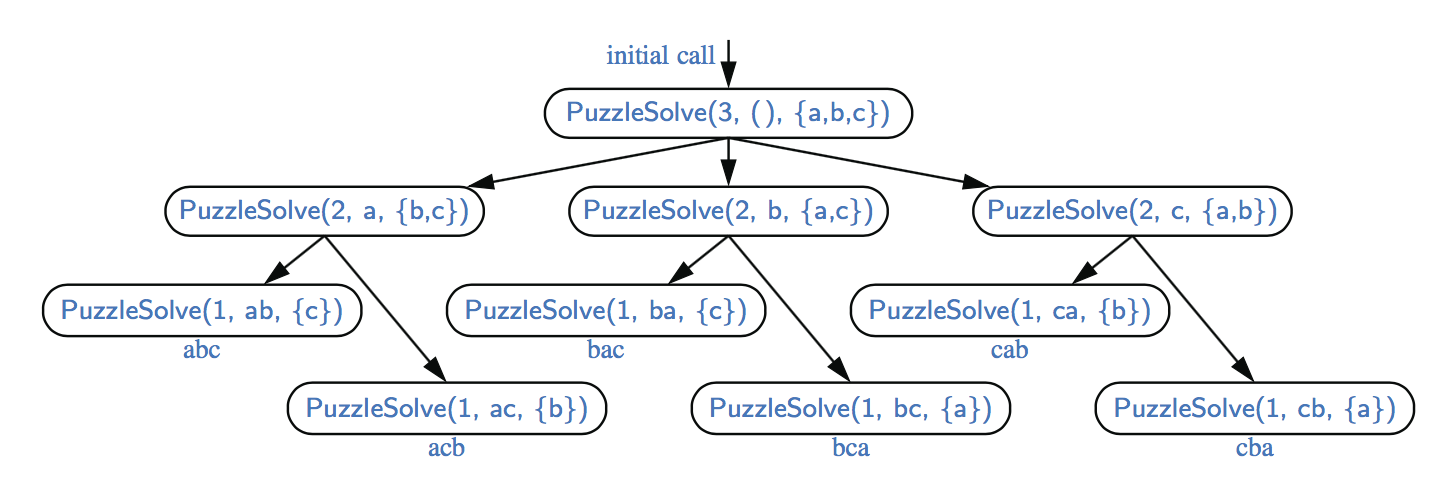• 基本情况测试。我们首先测试一组基本情况（至少应该有一个）。应该定义这些基本用例，以便每个可能的递归调用链最终都会到达一个基本用例，并且每个基本用例的处理不应该使用递归。
• 复发。如果不是基本情况，则执行一个或多个递归调用。此重复步骤可能涉及一个测试，该测试决定要进行几个可能的递归调用中的哪一个。我们应该定义每个可能的递归调用，以便它朝着一个基本情况前进。

## 参数化递归

``````/∗∗ Returns true if the target value is found in the data array. ∗/
public static boolean binarySearch(int[ ] data, int target) {
return binarySearch(data, target, 0, data.length − 1); // use parameterized version
}``````### 注册